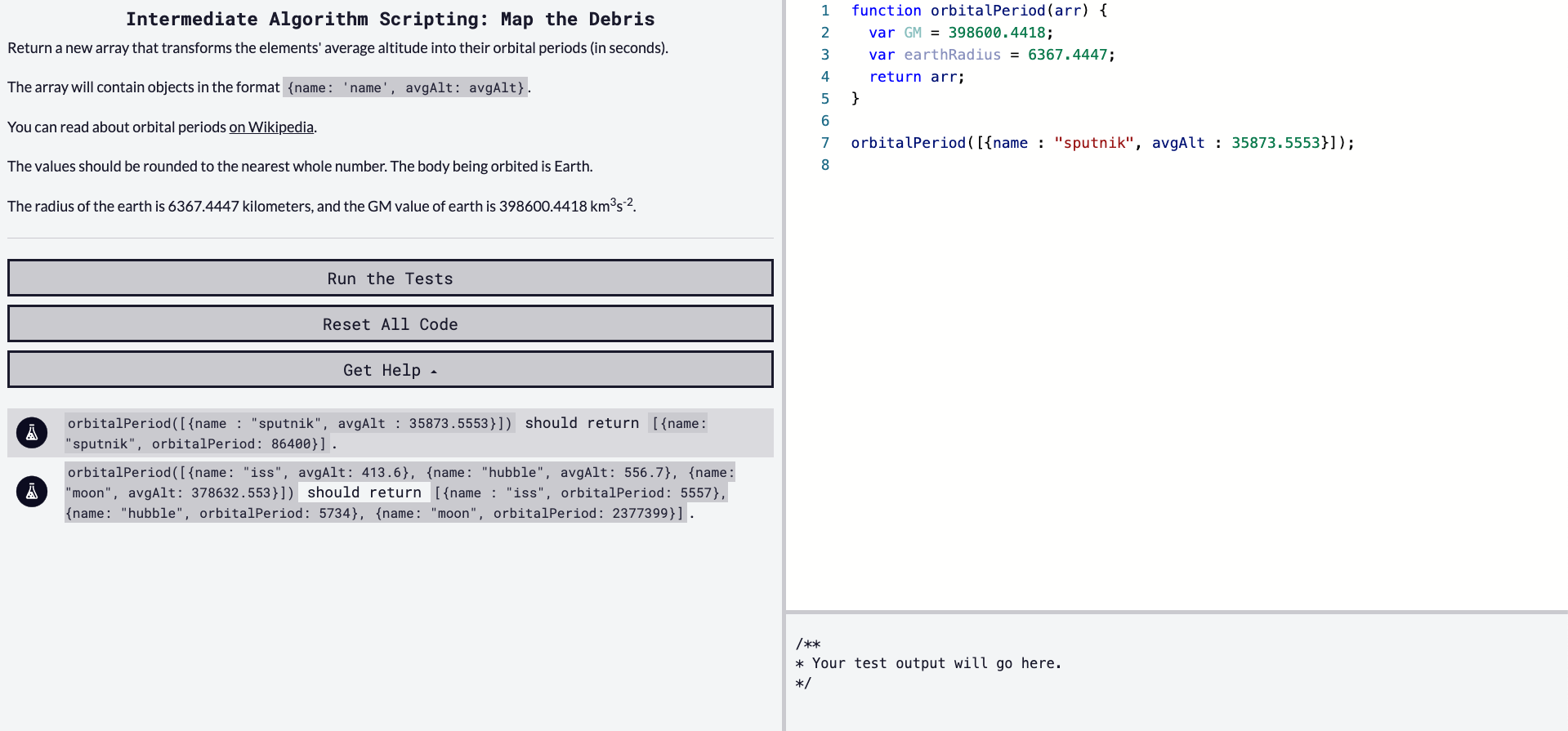## DEV Community is a community of 722,735 amazing developers

We're a place where coders share, stay up-to-date and grow their careers.

Viren B

Posted on • Originally published at virenb.cc

# Solving "Map the Debris" / freeCodeCamp Algorithm Challenges

** Post can also be found on virenb.cc **Let's solve freeCodeCamp's intermediate algorithm scripting challenge, 'Map the Debris'.

### Starter Code

``````function orbitalPeriod(arr) {
var GM = 398600.4418;
return arr;
}

orbitalPeriod([{name : "sputnik", avgAlt : 35873.5553}]);
``````

### Instructions

Return a new array that transforms the elements' average altitude into their orbital periods (in seconds).

The array will contain objects in the format `{name: 'name', avgAlt: avgAlt}`.

The values should be rounded to the nearest whole number. The body being orbited is Earth.

The radius of the earth is 6367.4447 kilometers, and the GM value of earth is 398600.4418 km3s-2.

### Test Cases

• `orbitalPeriod([{name : "sputnik", avgAlt : 35873.5553}])` should return `[{name: "sputnik", orbitalPeriod: 86400}]`.
• `orbitalPeriod([{name: "iss", avgAlt: 413.6}, {name: "hubble", avgAlt: 556.7}, {name: "moon", avgAlt: 378632.553}])` should return `[{name : "iss", orbitalPeriod: 5557}, {name: "hubble", orbitalPeriod: 5734}, {name: "moon", orbitalPeriod: 2377399}]`.

# Our Approach

The instructions for this challenge are short and to the point.

• Our input is an array of one or more objects.

• We have to return the same array of objects, but altering one of the key-value pairs (`avgAlt` -> `orbitalPeriod`).

• Make sure orbitalPeriod is calculated in seconds and rounded up to the nearest whole number.

The last challenge seems a bit hard. This formula is a little complex. But basically we have to do some math to calculate it, deleted `avgAlt`, and add in `orbitalPeriod` into each object in the array.

After a lot of Internet searching (formerly known as Googling - I don't like to feed into the search engine bias), I came across the correct formula, `T = 2 * pi * sqrt(r^3/GM); r = earthRadius + avgAlt`.

GM is provided in the instructions, as is the radius of the Earth. We can start by setting those variables.

``````const GM = 398600.4418;
``````

Now that we have our values and a formula, we can plug in those values and do some math. Once we have the orbital period value, we can add it to each object, and remove the `avgAlt`. We can loop over each object in the array using `map()`.

``````arr.map(obj => {
let oP = Math.round(2 * Math.PI * Math.sqrt(Math.pow(earthRadius + obj.avgAlt, 3) / GM));
// More stuff
})
``````

So we set a variable with the above formula. We use `Math.round()` as the instructions wanted us to round to the nearest whole number. Next we can delete `avgAlt` as we have used it in the formula. Then we can add `oP` to the object.

``````arr.map(obj => {
let oP = Math.round(2 * Math.PI * Math.sqrt(Math.pow(earthRadius + obj.avgAlt, 3) / GM));
// Delete avgAlt
delete obj.avgAlt;
obj.orbitalPeriod = op;
})
``````

We have updated the object(s) in `arr`. Lastly, we want to make sure to return `arr`.

# Our Solution

``````function orbitalPeriod(arr) {
var GM = 398600.4418;
arr.map(obj => {
let oP = Math.round(2 * Math.PI * Math.sqrt(Math.pow(earthRadius + obj.avgAlt, 3) / GM));
delete obj.avgAlt;
obj.orbitalPeriod = oP;
})
return arr;
}
``````